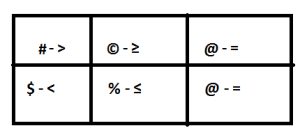#Coded Inequality Problems

Let’s solve some Coded Inequality Problems

Directions

P © Q means P is not smaller than Q

P % Q means P is not greater than Q

P # Q means P is neither smaller than nor equal to Q

P @ Q means P is neither greater than nor smaller than Q

P \$ Q means P is neither greater than nor equal to Q

Let’s form a table first.Problem 1

Statements F % T, T @ J, J # W.

Conclusions 1) J @ F.  2) J # F.  3) W \$ T.

Modified Statement: F % T @ J # W

Conclusions

1. J @ F – Reverse Line. Symbols are @ and %. Higher priority is %. Since it is Reverse line we should look the symbol opposite to %. i.e. ©. Conclusion 1 is False.

2. J # F – Reverse Line. Symbols are @ and %. Higher priority is %. Since it is Reverse line we should look the symbol opposite to %. i.e. ©. Conclusion 2 is False.

3. W \$ T – Reverse Line. Symbols are # and @. Both symbols are in Row 1. High priority goes to #. Since it is a reverse line, we should note the symbol opposite to #. i.e. \$. Conclusion 3 is True.

NOTE

If we look above, both conclusions 1 and 2 are false. Now check for Merging concept. They have similar characters. Both are false. Now try to combine or merge the symbols of the conclusions. If we merge @ and #, we will get ≥. So it forms a meaningful conclusion.

So, Only either 1 or 2 and 3 are true.

Problem 2

Statements – R # D, D © K, K \$ M.

Conclusions – 1. M # R.   2. K \$ R.   3. D # M

Modified Statement – R # D © K \$ MConclusions

1. M # R – Reverse Line. Symbols – \$, ©, and #. They are in different rows. FALSE

2. K \$ R – Reverse Line. Symbols – © and #. Both are in Row 1. # is higher priority. Symbol opposite to # is \$. So TRUE.

3. D # M – Forward line. Symbols – © and \$. Different Rows. So False.

We cannot apply merging concept here, as the characters are different.

Only Conclusion 2 is True

Problem 3

Statements – Z © F, F \$ M, M % K

Conclusions – 1) K # F.    2) Z # M.    3) K # Z.

Modified Statement: Z © F \$ M % KConclusions

1) K # F – Reverse Line. Symbols are % and \$. High Priority – \$. Symbol opposite to \$ is #. TRUE.

2) Z # M – Forward Line. Symbols are © and \$. Different Rows. FALSE

3) K # Z – Reverse Line. Symbols are %, \$, and ©. Different Rows. False.

Only Conclusion 1 is True.

Problem 4

Statement – H @ B, B © R, A \$ R

Conclusion – 1. B © A   2. R % H.   3. A \$ H

Modified Statement H @ B © R # A.

(In previous problems it was in a sequence and we easily combined the statements. When there is change in sequence, we should change the symbol and write it in a sequence.)

Here, Second statement is B © R and third Statement is A \$ R. To combine these two, we should change the third statement as R # A. (# is opposite to \$). Now we can combine them as B © R # A.

Modified Statement H @ B © R # AConclusions

1. B © A – Forward Line.  Symbols between B and A are © and #. High Priority is #. FALSE

2. R % H – Reverse Line. Symbols – © and @. High Priority for ©. Opposite to © is %. TRUE

3. A \$ H – Reverse Line. Symbols – #, ©, and @. High Priority for #. Symbol opposite to # is \$. So True.

Conclusions 2 and 3 are True.

NOTE: When a conclusion forms Forward line, just look for the highest priority symbol. When a conclusion forms Reverse Line, look for the symbol which is opposite to the highest priority symbol.

AffairsCloud Recommends Oliveboard Mock Test

AffairsCloud Ebook - Support Us to Grow